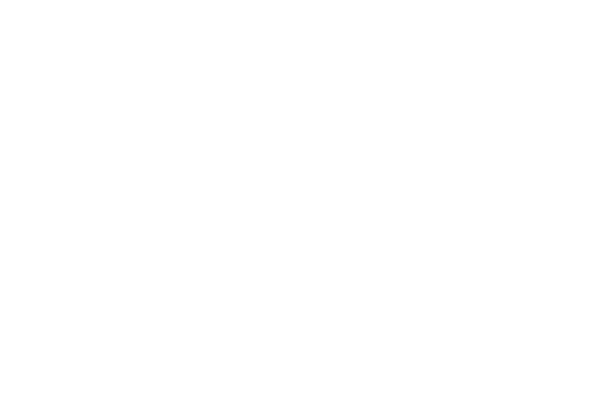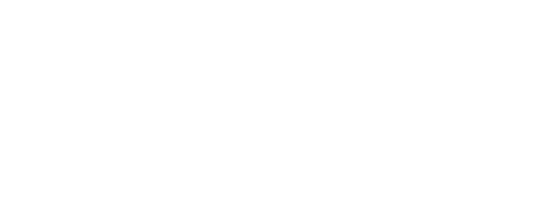# MIDI INTERFACE

### INTRODUCTION

The MIDI interface is basically a hi-speed serial interface running at 31,250 baud. There are 8 data bits wth 1 start and 1 stop bit. The dmmidi0 device basically provides simple read() and write() access to the MIDI device on the sound card.``````

### MIDI NOTE SPECIFICATION

The following table specifies the Note Pitch number. For FM synthesis, the octave simply is the Note Pitch Number divided by 12 (NP / 12) and the Frequency is Note Pitch Number is the Note Table modulo 12 (table[NP % 12]) where the Note Table is the table of Note Names to Frequencies described in the section on FM synthesizer Programming. See example code below.In order to read input from a MIDI synthesizer you need to loop on reading the status byte first. Depending on the type of the Status Byte, additional bytes are read from the MIDI keyboard. Reading data from the MIDI controller is achieved as follows:

• Open the Sound Blaster MIDI device using an open call. This returns a MIDI device file descriptor.
• Read one byte of Status Info. Use a read call on the MIDI device file descriptor to read 1 byte.
• Decode the Status byte using the table above. If the Status byte requires 2 data bytes then read two bytes of data into different variables (one byte at a time). If the Status byte requires 1 data byte then issue a single byte read.
• Perform the necessary operations on the data. (Send it to the FM synthesizer)
• Loop to Reading Status Info byte.

### READING FROM MIDI FILES USING MIDILIB

To read MIDI codes from MIDI files in Format 1 or 0 requires the use of the standard MIDIfile library which is supplied along with the software. Please read the documentation on reading MIDI files using the library. The following example (taken from the library example) demonstrates the scheme to read MIDI files and output music. MIDI files basically contain a header with track and SMPTE time code information. The header is also reponsible for the tempo of the MIDI tune. Following the header is a Track Start flag with SMPTE time codes followed by a MIDI opcode described above followed by two or three bytes of data depending on the MIDI opcode. The following scheme describes how to process MIDI files for output to the FM synthesizer or a MIDI synthesizer connected to the MIDI port.

First define your MIDI functions to perform the following basic MIDI codes:

• Key Off
• Key On
• Program Change
• Key Pressure
• Channel Pressure
• Pitch Wheel
• Controller Change (Mf_parameter)
• System Exclusive

These functions are called by the library and can be used to either hook into the FM synthesizer or ouput directly via a write to the MIDI port.

• From main(), you need to initialize all the MIDI library function pointers to point to your specific functions. Otherwise library defaults are taken. In most cases you will have to define the MIDI library function Mf_getc to point to a local "getc" type of function which reads a charater from the MIDI file and returns it to the calling function in the library. In order to define a "getc" type of routine, you will have to pass a file descriptor which is obtained using an fopen() system call.
• Issue a call to midifile() routine which reads the MIDI Format 1 files and parses the information.
• Close the open file descriptor.

The C-code below describes how to declare local functions. The only functions you need to define for parsing MIDI files are:

```        Mf_error = error;
Mf_starttrack =  txt_trackstart;
Mf_endtrack =  txt_trackend;
Mf_on =  txt_noteon;
Mf_off =  txt_noteoff;
Mf_pressure =  txt_pressure;
Mf_controller =  txt_parameter;
Mf_pitchbend =  txt_pitchbend;
Mf_program =  txt_program;
Mf_chanpressure =  txt_chanpressure;
Mf_sysex =  txt_sysex;
Mf_metamisc =  txt_metamisc;
Mf_seqnum =  txt_metaseq;
Mf_eot =  txt_metaeot;
Mf_timesig =  txt_timesig;
Mf_smpte =  txt_smpte;
Mf_tempo =  txt_tempo;
Mf_keysig =  txt_keysig;
Mf_sqspecific =  txt_metaspecial;
Mf_text =  txt_metatext;
Mf_arbitrary =  txt_arbitrary;```

These functions handle the basic MIDI codes described above as well as handle error, SMPTE and sysex codes found in mode MIDI Format 1 files. You may wish to ignore them if you need rudimentary MIDI capabilities. The following code uses an array to hold about 100000 MIDI events which are written to the MIDI port of the sound blaster. This array is sorted accroding to the SMPTE time code since MIDI files handle one track at a time. These functions handle the basic MIDI codes described above as well as handle error, SMPTE and sysex codes found in mode MIDI Format 1 files. You may wish to ignore them if you need rudimentary MIDI capabilities. The following code uses an array to hold about 100000 MIDI events which are written to the MIDI port of the sound blaster. This array is sorted accroding to the SMPTE time code since MIDI files handle one track at a time.

```/*
* mftext
*
* Convert a MIDI file to verbose text.
*/
#include <stdio.h>
#include <ctype.h>
#include <time.h>
#include <fnctl.h>
#include "midifile.h"

static FILE *F;
struct event {
char data0;
char data1;
char data2;
int time_stamp;
};

struct event midievent;
int current_event = 0;

main(argc,argv)
char **argv;
{
FILE *efopen();
int blastfd;

if ( argc > 1 )
F = efopen(argv,"r");
else
F = stdin;
blastfd = open("/dev/sbpmidi0", O_WRONLY);
/* Initialize the function pointer in the MIDI file library */
initfuncs();
Mf_getc = filegetc;

/* Start processing the MIDI file */
midifile();
fclose(F);
/* qsort the events based on the time stamp */
qsort(midievent, current_event, sizeof(event), compare);

/* write the events out to the MIDI port. Wait for the time on the event */
for (i=0; i<current_event; i++) {
waitfor(midi_event[i].time_stamp);
write(blastfd, midi_event[i].data0, 1);
write(blastfd, midi_event[i].data1, 1);
/* Write the 3 byte of data only if necessary */
if (midievent[i].bytes == 3)
write(blastfd, midi_event[i].data3, 1);
}
exit(0);
}

/* This is the getc routine to read the MIDI file */
filegetc()
{
return(getc(F));
}

/* This routine opens the MIDI file and flags errors */
FILE *
efopen(name,mode)
char *name;
char *mode;
{
FILE *f;
extern int errno;
extern char *sys_errlist[];
extern int sys_nerr;
char *errmess;
if ( (f=fopen(name,mode)) == NULL ) {
(void) fprintf(stderr,"*** ERROR *** Cannot open '%s'!\n",name);
if ( errno <= sys_nerr )
errmess = sys_errlist[errno];
else
errmess = "Unknown error!";
(void) fprintf(stderr,"************* Reason: %s\n",errmess);
exit(1);
}
return(f);
}

/* Compare two events -- used for the qsort c-library function */
int compare(event *one, event *two)
{
return (one->time_stamp - two->time_stamp)
}

/* suspend the program until the time is up for the event */
waitfor(long currtime)
{
static ulong last_event = 0;
struct timeval tv;
ulong usecs;

if (last_event == 0) {
last_event = currtime;
return;
}
usecs = currtime - last_event;
last_event = currtime;
tv.tv_sec = usecs/1000000;
tv.tv_usec = usecs % 1000000;
select(0,0,0,0, &tv);
}

/* print errors in the MIDI file */
error(s)
char *s;
{
fprintf(stderr,"Error: %s\n",s);
}

/* Print the header information regarding MIDI format, tracks and tempo */
{
}

txt_trackstart()
{
printf("Track start\n");
}

txt_trackend()
{
printf("Track end\n");
}

/* Handle key off events - Refer to MIDI opcode chart */
txt_noteon(chan,pitch,vol)
{
prtime();
printf("Note on, chan=%d pitch=%d vol=%d\n",chan+1,pitch,vol);
midievent[current_event].data0 = chan;
midievent[current_event].data1 = pitch;
midievent[current_event].data2 = vol;
midievent[current_event++].bytes = 3;
}

/* Handle key on events - Refer to MIDI opcode chart */
txt_noteoff(chan,pitch,vol)
{
prtime();
printf("Note off, chan=%d pitch=%d vol=%d\n",chan+1,pitch,vol);
midievent[current_event].data0 = chan;
midievent[current_event].data1 = pitch;
midievent[current_event].data2 = vol;
midievent[current_event++].bytes = 3;
}

/* Handle key pressure event - Refer to MIDI opcode chart */
txt_pressure(chan,pitch,press)
{
prtime();
printf("Pressure, chan=%d pitch=%d press=%d\n",chan+1,pitch,press);

}

/* Handle control change events - Refer to MIDI opcode chart */
txt_parameter(chan,control,value)
{
prtime();
printf("Parameter, chan=%d c1=%d c2=%d\n",chan+1,control,value);
midievent[current_event].data0 = chan;
midievent[current_event].data1 = control;
midievent[current_event++].data2 = value;
}

/* Handle pitch bend - Refer to MIDI opcode chart */
txt_pitchbend(chan,msb,lsb)
{
prtime();
printf("Pitchbend, chan=%d msb=%d lsb=%d\n",chan+1,msb,lsb);
midievent[current_event].data0 = chan;
midievent[current_event].data1 = msb;
midievent[current_event].data2 = lsb;
midievent[current_event++].bytes = 3;
}

/* Handle program change events - Refer to MIDI opcode chart */
txt_program(chan,program)
{
prtime();
printf("Program, chan=%d program=%d\n",chan+1,program);
midievent[current_event].data0 = chan;
midievent[current_event].data1 = program;
midievent[current_event++].bytes = 2;
}

/* Handle channel pressure events - refer to MIDI opcode chart */
txt_chanpressure(chan,press)
{
prtime();
printf("Channel pressure, chan=%d pressure=%d\n",chan+1,press);
midievent[current_event].data0 = chan;
midievent[current_event].data1 = press;
midievent[current_event++].bytes = 2;
}

/* Handle sysex events - Refer to MIDI opcode chart */
txt_sysex(leng,mess)
char *mess;
{
prtime();
printf("Sysex, leng=%d\n",leng);
}

/* Unrecognized meta events in the MIDI file - flag warnings */
txt_metamisc(type,leng,mess)
char *mess;
{
prtime();
printf("Meta event, unrecognized, type=0x%02x leng=%d\n",type,leng);
}

txt_metaspecial(type,leng,mess)
char *mess;
{
prtime();
printf("Meta event, sequencer-specific, type=0x%02x leng=%d\n",type,leng);
}

txt_metatext(type,leng,mess)
char *mess;
{
static char *ttype[] = {
NULL,
"Text Event",       /* type=0x01 */
"Sequence/Track Name",
"Instrument Name",  /* ...       */
"Lyric",
"Marker",
"Cue Point",            /* type=0x07 */
"Unrecognized"
};
int unrecognized = (sizeof(ttype)/sizeof(char *)) - 1;
register int n, c;
register char *p = mess;        if ( type < 1 || type > unrecognized
)
type = unrecognized;
prtime();
printf("Meta Text, type=0x%02x (%s)  leng=%d\n",type,ttype[type],leng);
printf("     Text = <");
for ( n=0; n<leng; n++ ) {
c = *p++;
printf( (isprint(c)||isspace(c)) ? "%c" : "\\0x%02x" , c);
}
printf(">\n");
}

txt_metaseq(num)
{
prtime();
printf("Meta event, sequence number = %d\n",num);
}

txt_metaeot()
{
prtime();
printf("Meta event, end of track\n");
}

txt_keysig(sf,mi)
{
prtime();
printf("Key signature, sharp/flats=%d  minor=%d\n",sf,mi);
}

txt_tempo(tempo)
long tempo;
{
prtime();
printf("Tempo, microseconds-per-MIDI-quarter-note=%d\n",tempo);
}

txt_timesig(nn,dd,cc,bb)
{
int denom = 1;
while ( dd-- > 0 )
denom *= 2;
prtime();
printf("Time signature=%d/%d  MIDI-clocks/click=%d  32nd-notes/24-MIDI-clocks=%d\n",
nn,denom,cc,bb);
}

/* Parse the SMPTE time stamp and print the information */
txt_smpte(hr,mn,se,fr,ff)
{
prtime();
printf("SMPTE, hour=%d minute=%d second=%d frame=%d fract-frame=%d\n",
hr,mn,se,fr,ff);
}

txt_arbitrary(leng,mess)
char *mess;
{
prtime();
printf("Arbitrary bytes, leng=%d\n",leng);
}

prtime()
{
printf("Time=%ld  ",Mf_currtime);
}

/* Initialize functions - refer to midifile.h for details */
initfuncs()
{
Mf_error = error;
Mf_starttrack =  txt_trackstart;
Mf_endtrack =  txt_trackend;
Mf_on =  txt_noteon;
Mf_off =  txt_noteoff;
Mf_pressure =  txt_pressure;
Mf_controller =  txt_parameter;
Mf_pitchbend =  txt_pitchbend;
Mf_program =  txt_program;
Mf_chanpressure =  txt_chanpressure;
Mf_sysex =  txt_sysex;
Mf_metamisc =  txt_metamisc;
Mf_seqnum =  txt_metaseq;
Mf_eot =  txt_metaeot;
Mf_timesig =  txt_timesig;
Mf_smpte =  txt_smpte;
Mf_tempo =  txt_tempo;
Mf_keysig =  txt_keysig;
Mf_sqspecific =  txt_metaspecial;
Mf_text =  txt_metatext;
Mf_arbitrary =  txt_arbitrary;
}

```